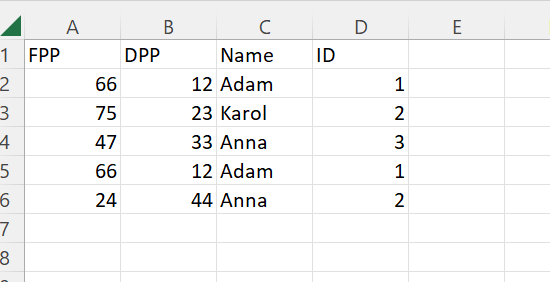# Queue item, unique References

Hello,

I want to add unique items to the queue.
I wanted to use References, not only one value but several
I have a DT, and References should be a combination of FPP,DPP,NAME,ID
Can you advise how to modify Reference sectionTest.zip (3.4 KB)
Test.xlsx (8.8 KB)

I believe you would require to keep the value in the below way :

``````CurrentRow("FPP").ToString+CurrentRow("DPP").ToString+CurrentRow("Name").ToString+CurrentRow("ID").ToString
``````

In the reference section you can do like @supermanPunch suggested

If you want comma or space in between your combination

For Space

``````CurrentRow("FPP").ToString+" "+CurrentRow("DPP").ToString+" "+CurrentRow("Name").ToString+" "+CurrentRow("ID").ToString
``````

For Comma

``````CurrentRow("FPP").ToString+","+CurrentRow("DPP").ToString+","+CurrentRow("Name").ToString+","+CurrentRow("ID").ToString
``````

Regards
Sudharsan

in general as mentioned above, but we can reduce the redundant code parts by:

``````String.Join("_",{"FFP","DDP","Name","ID"}.Select(Function (x) CurrentRow(x).toString.Trim))
``````